# Logarithm

Parent topic:
Arithmetic
•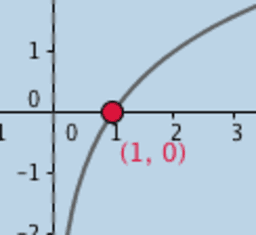### Logarithmic Functions: Graphs

Activity

Tim Brzezinski

•### Derivative of Natural Log Function

Activity

Tim Brzezinski

•### Logarithmic Action (4)!

Activity

Tim Brzezinski

•### Logarithmic Action (3)!

Activity

Tim Brzezinski

•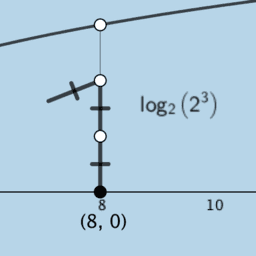### Logarithmic Action (4)! V2

Activity

Tim Brzezinski

•### Logarithmic Action (3: V2)

Activity

Tim Brzezinski

•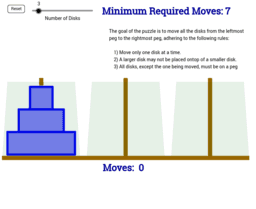### Games

Book

Manuel Graf

•### The touches of y=a^x and y = log{a} x

Activity

Anthony OR 柯志明

•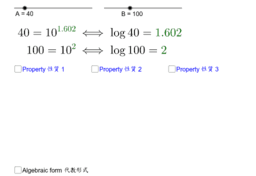### Properties of Logarithms 對數的性質

Activity

Alex CHIK

•### Solving Exponential Equation

Activity

Arthur Lee

•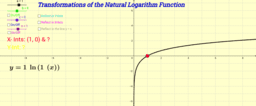### Transforming Natural Logarithm Function

Activity

Andy Wain

•Book

Arthur Lee

•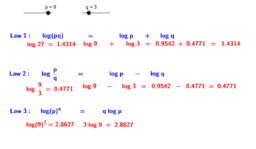### Law of Logarithms

Activity

Hisham Amir

•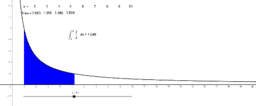### Area under 1/x and the natural logarithm function

Activity

Bernard Murphy

•### Exponential Equation

Activity

Arthur Lee

•### Natural Logarithm as an Integral

Activity

Steve Phelps

•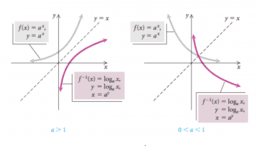### Exploring Exponential & Logarithmic Functions

Book

Mina Sedaghatjou

•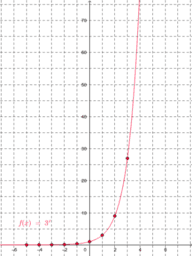### Geom. & Alg.: Exponential Functions & Logarithms

Book

Terry Lee Lindenmuth

•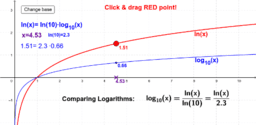### Comparing logarithms

Activity

Linda Fahlberg-Stojanovska

•### TURKEY (SAMSUN): Logarithm.

Activity

GülseminTuran

•### Logarithm function explorer

Activity

Audrey McLaren

•### Using Logarithm laws to solve equations

Activity

Hisham Amir

•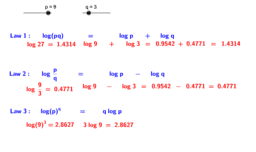### Laws of Logarithms

Activity

Folens Publishers

•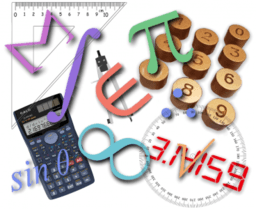Book